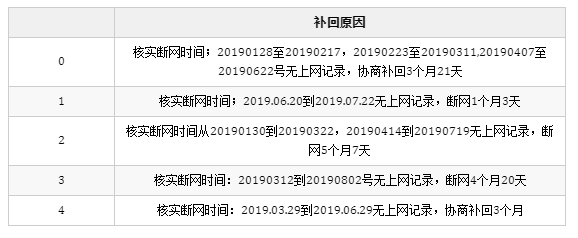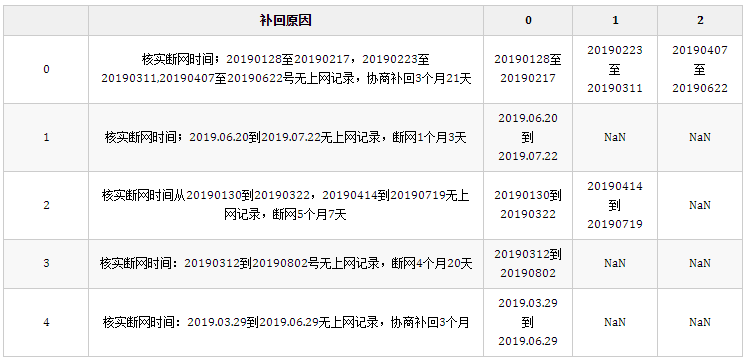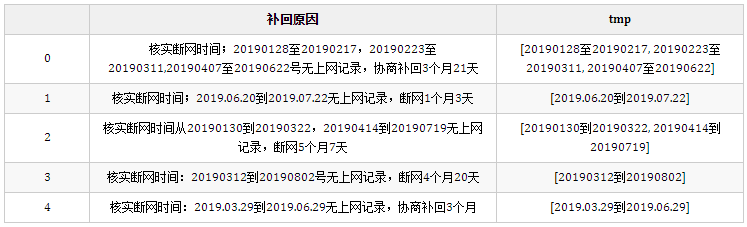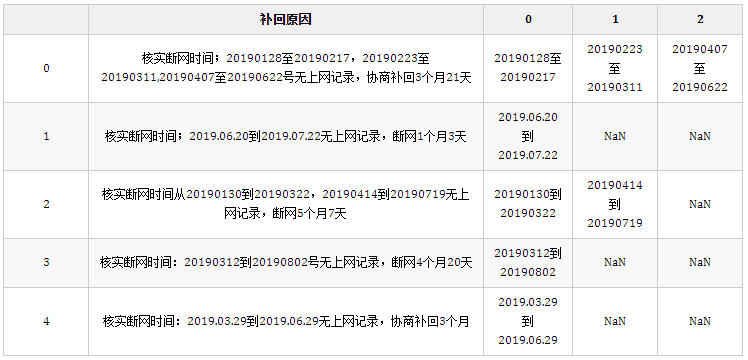## 2.解法

#### 2.1 基础解法explode函数

```import pandas as pd

mydict = {'A': , 'B': [2, 3], 'C': [4, 5, 6]}
pd.DataFrame(mydict.items()).explode(1)```

`mydict.items()`是python基础字典的内容，它返回了这个字典键值对组成的元组列表：

`mydict.items()`

`dict_items([('A', ), ('B', [2, 3]), ('C', [4, 5, 6])])`

`pd.DataFrame(mydict.items())`

`select a,b_i from df lateral view explode(b) tmp as b_i;`

#### 2.3 循环法解题

```result = []
for k, vs in mydict.items():
for v in vs:
result.append((k, v))
pd.DataFrame(result)```

```import itertools
result = []
for k, v in mydict.items():
result.extend(itertools.product(k, v))
pd.DataFrame(result)```

`product(*iterables, repeat=1) --> product object`

• iterables 为可迭代对象
• 可选参数repeat 表示重复次数

• product(‘ab’, range(3)) –> (‘a’,0) (‘a’,1) (‘a’,2) (‘b’,0) (‘b’,1) (‘b’,2)
• product((0,1), (0,1), (0,1)) –> (0,0,0) (0,0,1) (0,1,0) (0,1,1) (1,0,0) …

```result = [(k, v) for k, vs in mydict.items() for v in vs]
pd.DataFrame(result)```

#### 2.4 变形法解题

```df = pd.DataFrame(mydict.items(), columns=["a", "b"])
df```

#### 对Series进行列表分列

`df["b"].apply(pd.Series)`

`df.set_index("a")["b"].apply(pd.Series)`

`df["b"].apply(pd.Series).set_index(df["a"])`

#### 直接对Datafream进行列表分列

`df.agg({"a": lambda x: x, "b": pd.Series})`

`df.agg({"a": lambda x: x, "b": pd.Series}).droplevel(0, axis=1)`

`df.agg({"a": lambda x: x, "b": pd.Series}).droplevel(0, axis=1).set_index("a")`

#### 2.4.2 将字典的键作为索引的2种读取方法

`df = pd.DataFrame.from_dict(mydict, 'index')`

`df = pd.DataFrame(data=mydict.values(), index=mydict.keys())`

#### 2.4.3 melt实现逆透视

```df = df.rename_axis(index="a").reset_index()
df```

`df.melt(id_vars='a', value_name='b')`

```df = pd.DataFrame.from_dict(mydict, 'index')
df = df.melt(id_vars='a', value_name='b').drop(columns="variable").dropna()
df.b = df.b.astype("int")
df```

#### 2.4.4 stack实现逆透视

```df = pd.DataFrame.from_dict(mydict, 'index')
df.stack()```

```A  0    1.0
B  0    2.0
1    3.0
C  0    4.0
1    5.0
2    6.0
dtype: float64```

`df.stack().droplevel(1)`

```A    1.0
B    2.0
B    3.0
C    4.0
C    5.0
C    6.0
dtype: float64```

`df.stack().droplevel(1).reset_index()`

`df.stack().droplevel(1).reset_index().set_axis(["a", "b"], axis=1)`

`df.b = df.b.astype("int")`

```df = pd.DataFrame.from_dict(mydict, 'index')
df = df.stack().droplevel(1).reset_index().set_axis(["a", "b"], axis=1)
df.b = df.b.astype("int")
df```

## 2.实际应用

``import pandas as pdpd.set_option("display.max_colwidth", 100)``

## 正则提取并分列

``df = pd.read_excel("正则提取与分列.xlsm", usecols=)df.head()````result = df.copy()result["tmp"] = result["补回原因"].str.findall("([\d.]+[到至][\d.]+)")result = result.agg({"补回原因": lambda x: x, "tmp": pd.Series}).droplevel(0, axis=1)result.head()````df["tmp"] = df["补回原因"].str.findall("([\d.]+[到至][\d.]+)")df.head(5)````df.agg({"补回原因": lambda x: x, "tmp": pd.Series})``

``df.agg({"补回原因": lambda x: x, "tmp": pd.Series}).droplevel(0, axis=1).head()```droplevel(0, axis=1)`用于删除多级索引指定的级别，axis=0可以删除行索引，axis=1则可以删除列索引，第一参数表示删除级别0。当然如果列索引存在名称时还可以传入名称字符串，可参考官网文档：

``df = pd.DataFrame([...     [1, 2, 3, 4],...     [5, 6, 7, 8],...     [9, 10, 11, 12]... ]).set_index([0, 1]).rename_axis(['a', 'b'])>>> df.columns = pd.MultiIndex.from_tuples([...    ('c', 'e'), ('d', 'f')... ], names=['level_1', 'level_2'])>>> dflevel_1   c   dlevel_2   e   fa b1 2      3   45 6      7   89 10    11  12>>> df.droplevel('a')level_1   c   dlevel_2   e   fb2        3   46        7   810      11  12>>> df.droplevel('level2', axis=1)level_1   c   da b1 2      3   45 6      7   89 10    11  12``

## 分组聚合并分列

``df = pd.read_excel("分组聚合并分列.xlsx")df``

``(    df.groupby("姓名")["得分"]    .apply(list)    .apply(pd.Series)    .fillna("")    .rename(columns=lambda x: f"得分{x+1}")    .reset_index()    .astype({"得分1":"int8"}))``

``df.groupby("姓名")["得分"].apply(list)``

``姓名孙四娘          [7, 28]看见星光    [88, 28, 23]看见月光    [69, 10, 87]老祝          [51, 29]马青梅             Name: 得分, dtype: object``

``df.groupby("姓名")["得分"].apply(lambda x:x.to_list())``

``_.apply(pd.Series)``

``_.fillna("")``

fillna表示填充缺失值，传入””表示将缺失值填充为空字符串。

``_.rename(columns=lambda x: f"得分{x+1}")``

``_.reset_index()``

``_.astype({"得分1":"int8"})``

## 解析json字符串并字典分列

``df = pd.read_excel("字典分列.xlsx")df.head()``

``result = df.features.apply(eval).apply(pd.Series)result["counts"] = df.countsresult``

储存条件 品牌 推荐理由 品种 食用方式 是否进口 特色服务 是否有机 counts
0 常温 NaN NaN NaN NaN NaN NaN NaN 33
1 冷藏 NaN NaN NaN NaN NaN NaN NaN 24
2 常温 禾煜 NaN NaN NaN NaN NaN NaN 22
3 常温 妙洁 NaN NaN NaN NaN NaN NaN 16
4 冷冻 NaN NaN NaN NaN NaN NaN NaN 14
2083 常温 乐事 够薄够脆 NaN NaN NaN NaN NaN 1
2084 冷藏 NaN 生态种植 黄瓜 NaN NaN NaN 有机 1
2085 冷藏 NaN 腥味较淡 鲫鱼 NaN NaN 免费宰杀 NaN 1
2086 冷藏 NaN 甜脆可口 佛手瓜 NaN NaN NaN NaN 1
2087 冷藏 叮咚日日鲜 全程可追溯 猪小排 NaN NaN NaN NaN 1

2088 rows × 9 columns

`df.features.apply(eval)`用于将features列的每个json字符串解析为字典对象。

`**.apply(pd.Series)`则可以将每个字典对象转换成Series，则可以将该字典扩展到多列，并将原始的Series转换为Datafream。

`result["counts"] = df.counts`则将原始数据的counts列添加到结果列中。

​Python实用宝典 ( pythondict.com )